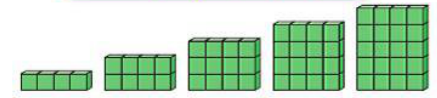##### Equations and Inequalities

+1
Set Details Share
created 7 years ago by fmarlatt
53 views
updated 7 years ago by fmarlatt
Subjects:
math
Page to share:
Embed this setcancel
COPY
code changes based on your size selection
Size:
X
1

Rebecca has more money than Jarrod.
How much more money does she have?

R - J

2

Jarrod and Rebecca picked up shells. How
many shells do they have in all?

R + J

3

Rebecca is twice as old as Jarrod.
How old is Rebecca?

R = 2xJ

4

Rebecca earned some money doing chores,
then gave 1/3 of her earnings to Jarrod.
How much did Jarrod have?

R ÷ 3 = J

5

Rebecca gave \$3 to Jarrod.
How much money did she have left?

R - 3

6

Rebecca and Jarrod combined the
money they earned for 2 weeks.
How much money did they
have in all?

(R + J) x 2

7

Rebecca read 3 times as many hours.
How many hours did Rebecca read?

R = 3 x J

8

Rebecca and Jarrod combined their money and
spent half on candy.
How much money did they
spend on candy?

(R + J) ÷ 2

9

Rebecca ran some laps around the track.
Jerrod ran 3 times as many laps.
How many did Jarrod run?

J = 3xR

10

Rebecca earned a certain score on her test.
Jarrod’s score was 3 points less than hers.
What was Jarrod’s score?

R - 3

11

r subtracted from 47

47 - r

12

j decreased by 47

j - 47

13

s + 47

14

r reduced by 47

r - 47

15

the sum of j and 47

j + 47

16

j less than 47

47 - j

17

the total of r and 47

r + 47

18

w more than 47

47 + w

19

47 fewer than w

w - 47

20

47 plus s

47 + s

21

the difference between 47 and s

47 - s

22

47 less j

j - 47

23

47 minus r

47 - r

24

twice R equals J

2 x R = J

25

half of J equals R

J ÷2 = R

26

R is three times as many as J

R = 3 x J

27

R is greater than J

R > J

28

J is greater than R

J > R

29

R is less than J

R< J

30

J is less than R

J < R

31

J plus 4 is greater than R

J + 4 > R

32

J plus 4 is less than R

J + 4 < R

33

the next number is always 6 less
than the number before it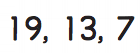34

the next number is always 6 more
than the number before it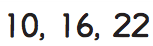35

the next number is always 9 less
than the number before it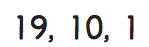36

the next number is always 9 more
than the number before it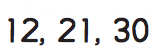37

the next number is always 6 less
than the number before it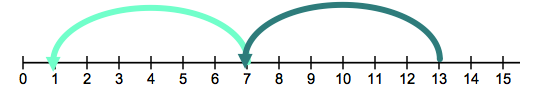38

the next number is always 4 more
than the number before it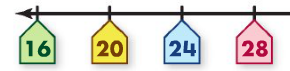39

the next number is always 6 more
than the number before it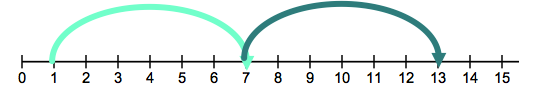40

the next number is always 3 less
than the number before it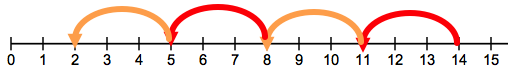41

the next number is always 5 more
than the number before it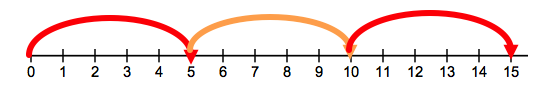42

the next shape has 1 less row than
than the shape before it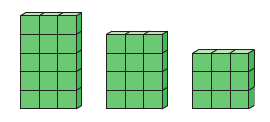43

the next shape has 1 more row than
than the shape before it